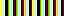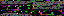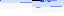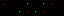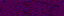```<?php
namespace entities\evr\security;

class Key_Hashes
{
public function __construct()
{
\$this->set_path();
\$this->set_hashes();
}
private function set_path()
{
\$this->path = \$GLOBALS["KEY_HASHES_PATH"];
}
private function set_hashes()
{
\$this->hashes = file(\$this->path, FILE_IGNORE_NEW_LINES);
}
public function write()
{
\$string = implode("\n", \$this->hashes) . "\n";
file_put_contents(\$this->path, \$string);
}
public function remove(\$hash)
{
\$hashes = \$this->hashes;
foreach (range(0, count(\$hashes) - 1) as \$ii)
{
if (\$hashes[\$ii] == \$hash)
{
array_splice(\$hashes, \$ii, 1);
break;
}
}
\$this->hashes = \$hashes;
}
}
```
```<?php
namespace entities\evr\security;
use entities\html as html;

class Security
{
public function __construct()
{
\$this->registrar = new Registrar();
}
{
{
}
{
}
}
{
\$action = \$this->get_post_parameter("action");
}
public function register_user()
{
return \$this->registrar->register();
}
public static function validate_key()
{
\$hashes = new Key_Hashes();
\$key = self::get_post_parameter("key");
if (self::find_hash(\$hashes, \$key))
{
return true;
}
return false;
}
public static function find_hash(\$hashes, \$key)
{
foreach (\$hashes->hashes as \$hash)
{
if (self::match_to_hash(\$key, \$hash))
{
return \$hash;
}
}
}
public static function match_to_hash(\$key, \$hash)
{
if (!\$hash)
{
return \$hash == crypt(\$key);
}
return \$hash == crypt(\$key, \$hash);
}
public static function show_error(\$message)
{
echo new html\Div(null, "error", \$message);
}
public function validate_user()
{
\$action = \$this->get_post_parameter("action");
{
return \$this->log_user_in();
}
{
}
}
public static function get_post_parameter(\$name)
{
if (isset(\$_POST[\$name]))
{
return \$_POST[\$name];
}
}
private function log_user_in()
{
{
if (\$this->get_post_parameter("remember"))
{
}
return true;
}
return false;
}
{
{
return \$hash;
}
}
{
\$root = \$GLOBALS["USERS_PATH"] . "/";
if (is_file(\$path))
{
return trim(file_get_contents(\$path));
}
return null;
}
{
\$root = \$GLOBALS["USERS_PATH"];
foreach (scandir(\$root) as \$file_name)
{
\$path = "\$root/\$file_name";
{
return \$file_name;
}
}
}
{
if (\$hash == \$stored)
{
{
return true;
}
}
return false;
}
public static function show_success(\$message)
{
echo new html\Div(null, "success", \$message);
}
public static function remove_key()
{
\$key = self::get_post_parameter("key");
\$hashes = new Key_Hashes();
\$hash = self::find_hash(\$hashes, \$key);
\$hashes->remove(\$hash);
\$hashes->write();
}
{
}
{
}
}
```
35.175.107.142
35.175.107.142
35.175.107.142

September 13, 2013

```from array import array
from time import sleep

import pygame
from pygame.mixer import Sound, get_init, pre_init

class Note(Sound):

def __init__(self, frequency, volume=.1):
self.frequency = frequency
Sound.__init__(self, self.build_samples())
self.set_volume(volume)

def build_samples(self):
period = int(round(get_init() / self.frequency))
samples = array("h",  * period)
amplitude = 2 ** (abs(get_init()) - 1) - 1
for time in xrange(period):
if time < period / 2:
samples[time] = amplitude
else:
samples[time] = -amplitude
return samples

if __name__ == "__main__":
pre_init(44100, -16, 1, 1024)
pygame.init()
Note(440).play(-1)
sleep(5)
```

This program generates and plays a 440 Hz tone for 5 seconds. It can be extended to generate the spectrum of notes with a frequency table or the frequency formula. Because the rewards in Send are idealized ocean waves, they can also be represented as tones. Each level has a tone in its goal and a tone based on where the player's disc lands. Both play at the end of a level, sounding harmonic for a close shot and discordant for a near miss. The game can dynamically create these tones using the program as a basis.

I'm also building an algorithmically generated song: Silk Routes (Scissored). Here is an example of how it sounds so far.